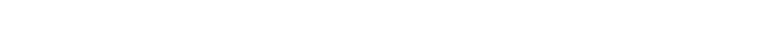# MIDI status & data bytes by Matt OttewillComputers are limited to communicating with the binary system of 0’s and 1’s. Computer microchips comprise vast numbers of switches (transistors) each of which may be either open (representing a 0) or closed (representing a 1). Here is a quick re-cap of relevant theory.

### BIT (binary digit)

Bit = A single value (either 0 or 1) is known as a binary digit or bit.

### Byte

Byte= As letters of the alphabet may be grouped together to form words, so bits may be grouped together to represent larger numbers. 8 bits together form a byte.### Most & least significant bits

MSB = The far left bit of a byte is known as the Most Significant Bit.

LSB =The far right bit of a byte is known as the Least Significant Bit.

### Nibble

Nibble = Bytes are sometimes broken down into two sets of four numbers each of which is known as a nibble.

# Status & data bytes

MIDI is an 8-bit language. It takes the form of Messages (or Commands) each of which are made up from a number of bytes.

There are two categories of byte in the MIDI language ...

1 Status bytes

2 Data bytes

The MSB of a byte in the MIDI language is used solely to indicate whether a byte is a Status or Data byte.

## Status Bytes

A MSB value of 1 indicates a Status byte.

The smallest number possible for a Status byte is 10000000 = 128 or (80H in hex).
The biggest number possible is 11111111 = 255 (or FFH in hex).

Thus the possible range of values a Status byte can be is 128-255.

### 1st nibble

The first four bits (nibble) indicate the type of message being sent. eg Note on or pitchbend.

### 2nd nibble

The last four bits indicate the MIDI channel on which the MIDI message is being sent. Four bits can represent values from 0-15 (or 16 separate values) which explains why we only have 16 MIDI channels.## Data Bytes

A MSB value of 0 indicates a Data byte.

The smallest number possible is 00000000 = 0 = 00H.
The biggest number possible is 01111111 = 127 = 7FH.

Thus the possible range of values a Data byte can be is 0-127. This explains why many MIDI controllers, such as volume or velocity, have a maximum value of 127.

# MIDI message example

Here is an example of a simple 3 byte MIDI message comprising a Status byte and 2 Data bytes. This message is telling a sound module set to respond on MIDI channel 1 to start playing a note (C3) at a velocity of 101.